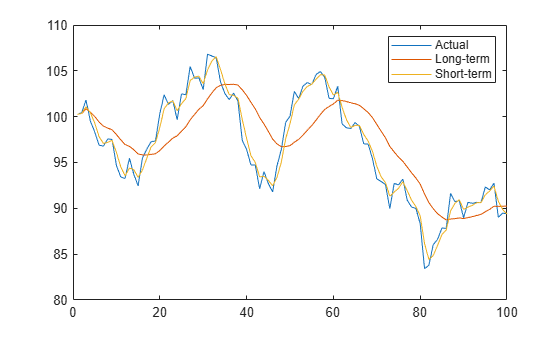# movavg

Moving average of a financial time series

`movavg` is updated to accept data input as a matrix, `table`, or `timetable`.

The syntax for `movavg` has changed. There is no longer support for the input arguments `Lead` and `Lag`, only a single `windowSize` is supported, and there is only one output argument (`ma`). If you want to compute the leading and lagging moving averages, you need to run `movavg` twice and adjust the `windowSize`.

## Syntax

``ma = movavg(Data,type,windowSize)``
``ma = movavg(___,Initialpoints)``
``ma = movavg(Data,type,weights)``
``ma = movavg(___,Initialpoints)``

## Description

example

````ma = movavg(Data,type,windowSize)` computes the moving average (MA) of a financial time series.```

example

````ma = movavg(___,Initialpoints)` adds an optional argument for `Initialpoints`. ```

example

````ma = movavg(Data,type,weights)` computes the moving average (MA) of a financial time series using a `'custom'` `type` and `weights`.```

example

````ma = movavg(___,Initialpoints)` adds an optional argument for `Initialpoints`. ```

## Examples

collapse all

Load the file `SimulatedStock.mat`, which provides a timetable (`TMW`) for financial data.

```load SimulatedStock.mat type = 'linear'; windowSize = 14; ma = movavg(TMW_CLOSE,type,windowSize)```
```ma = 1000×1 100.2500 100.3433 100.8700 100.4916 99.9937 99.3603 98.8769 98.6364 98.4348 97.8491 ⋮ ```

Load the file `SimulatedStock.mat`, which provides a timetable (`TMW`) for financial data.

```load SimulatedStock.mat type = 'linear'; malag=movavg(TMW_CLOSE,type,20) % Lagging moving average```
```malag = 1000×1 100.2500 100.3423 100.8574 100.4943 100.0198 99.4230 98.9728 98.7509 98.5688 98.0554 ⋮ ```
`malead=movavg(TMW_CLOSE,type,3) % Leading moving average`
```malead = 1000×1 100.2500 100.3580 101.0900 100.4300 99.3183 97.8217 97.0833 97.1950 97.4133 96.1133 ⋮ ```

Plot the leading and lagging moving averages.

```plot(TMW_CLOSE(1:100)) hold on plot(malead(1:100)) plot(malag(1:100)) hold off legend('Actual','Lead','Lag')```## Input Arguments

collapse all

Data for a financial series, specified as a column-oriented matrix, table, or timetable. Timetables and tables must contain variables with only a numeric type.

Data Types: `double` | `table` | `timetable`

Type of moving average to compute, specified as a character vector or string with an associated value.

Data Types: `char` | `string`

Number of observations of the input series to include in moving average, specified as a scalar positive integer. The observations include (`windowSize` - 1) previous data points and the current data point.

Note

The `windowSize` argument applies only to moving averages whose `type` is `'simple'`, `'square-root'`, `'linear'`, `'square'`, `'exponential'`, `'triangular'`, or `'modified'`.

Data Types: `double`

Custom weights used to compute the moving average, specified as a vector.

Note

The length of weights (N) determines the size of the moving average window (`windowSize`). The `weights` argument applies only to a `'custom'` `type` of moving average.

To compute moving average with custom weights, the weights (`w`) are first normalized such that they sum to one:

`W(i) = w(i)/sum(w), for i = 1,2,...,N`

The normalized weights (`W`) are then used to form the N-point weighted moving average (y) of the input Data (x):

```y(t) = W(1)*x(t) + W(2)*x(t-1) + ... + W(N)*x(t-N)```

The initial moving average values within the window size are then adjusted according to the method specified in the name-value pair argument `Initialpoints`.

Data Types: `double`

(Optional) Indicates how the moving average is calculated at initial points (before there is enough data to fill the window), specified as a character vector or string using one of the following values:

• `'shrink'` - Initializes the moving average such that the initial points include only observed data

• `'zero'` - Initializes the initial points with `0`

• `'fill'` - Fills initial points with `NaN`s

Note

The `Initialpoints` argument applies to all `type` specifications except for the `'exponential'` and `'modified'` options.

Data Types: `char` | `string`

## Output Arguments

collapse all

Moving average series, returned with the same number of rows (`M`) and the same type (matrix, table, or timetable) as the input `Data`.

 Achelis, S. B. Technical Analysis from A to Z. Second Edition. McGraw-Hill, 1995, pp. 184–192.